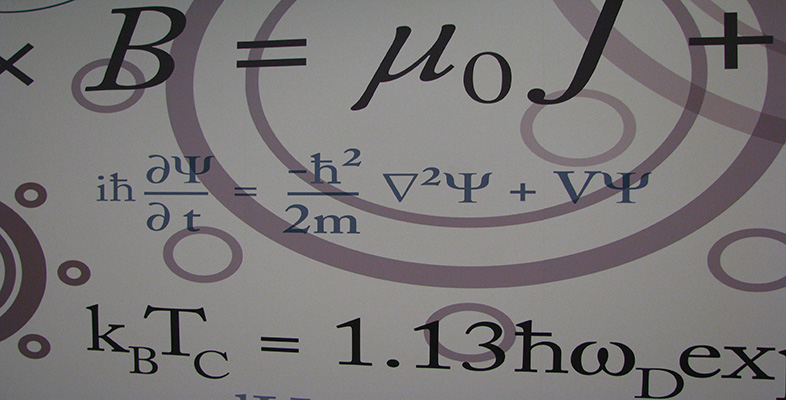Number systems

This free course is available to start right now. Review the full course description and key learning outcomes and create an account and enrol if you want a free statement of participation.

Free course

2.7 Arithmetical properties of complex numbers

The set of complex numbers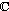satisfies all the properties previously given for arithmetic in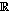. We state (but do not prove) these properties here.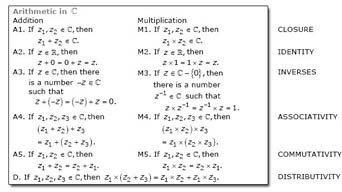In particular, 0 = 0 + 0i plays the same role inas the real number 0 does in, and 1 = 1 + 0i plays the same role as 1. These numbers are called identities for addition and multiplication respectively.

We also have that the additive inverse (or negative) of z = x + yi is −z = −xyi, and the multiplicative inverse (or reciprocal) of z = x + yi is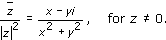There is one important difference between the set of real numbers and the set of complex numbers, however; namely that, unlike the real numbers, the complex numbers are not ordered.

For any two real numbers a and b, exactly one of the three properties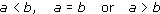is true. But this is not the case for the complex numbers; we cannot say, for example, that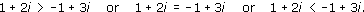Inequalities involving complex numbers make sense only if they are inequalities between real quantities, such as the moduli (moduli is the plural of modulus) of the complex numbers. For example, inequalities such as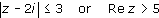are valid.

M208_6